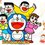# Need help for Recurrence!

Hello! Any hints for this? (Characteristic eqn doesn't seem to work for me here...)

$a_n = (n-1) a_{n-1} +n$.Note by Happy Melodies
7 years, 2 months ago

This discussion board is a place to discuss our Daily Challenges and the math and science related to those challenges. Explanations are more than just a solution — they should explain the steps and thinking strategies that you used to obtain the solution. Comments should further the discussion of math and science.

When posting on Brilliant:

• Use the emojis to react to an explanation, whether you're congratulating a job well done , or just really confused .
• Ask specific questions about the challenge or the steps in somebody's explanation. Well-posed questions can add a lot to the discussion, but posting "I don't understand!" doesn't help anyone.
• Try to contribute something new to the discussion, whether it is an extension, generalization or other idea related to the challenge.

MarkdownAppears as
*italics* or _italics_ italics
**bold** or __bold__ bold
- bulleted- list
• bulleted
• list
1. numbered2. list
1. numbered
2. list
Note: you must add a full line of space before and after lists for them to show up correctly
paragraph 1paragraph 2

paragraph 1

paragraph 2

[example link](https://brilliant.org)example link
> This is a quote
This is a quote
    # I indented these lines
# 4 spaces, and now they show
# up as a code block.

print "hello world"
# I indented these lines
# 4 spaces, and now they show
# up as a code block.

print "hello world"
MathAppears as
Remember to wrap math in $$ ... $$ or $ ... $ to ensure proper formatting.
2 \times 3 $2 \times 3$
2^{34} $2^{34}$
a_{i-1} $a_{i-1}$
\frac{2}{3} $\frac{2}{3}$
\sqrt{2} $\sqrt{2}$
\sum_{i=1}^3 $\sum_{i=1}^3$
\sin \theta $\sin \theta$
\boxed{123} $\boxed{123}$

Sort by:

You have to do plug and chug

This is called plug :

$a_n = (n-1)((n-2)a_{n-2}+n-1) + n$

This is called chug

$a_n = (n-1)(n-2)a_{n-2}+(n-1)^{2}+n$

Continue doing this until you arrive at a base case....You can see the general form after a few chugs. which you can prove by induction....

- 7 years, 2 months ago

I don't think there is an easy way to do this. Clearly there is no characteristic polynomial as the coefficients are variable. I plugged it into wolfram alpha after working on it to no avail and its general form is $a_n=2e\Gamma(n,1)-1$ where $a_1=1$.

$\Gamma(a,x)$ is the Incomplete Gamma Function which I have no clue of its definition.

- 7 years, 2 months ago

My original strategy was to substitute $b_n=a_{n+1}-c_1a_n$ for some constant $c$ such that everything cancels out neatly, but it got really messy and I ended up having $c_1=\dfrac{-n+\sqrt{n^2-4}}{2}$ (which is almost certainly wrong) then I stopped.

- 7 years, 2 months ago

Yes me too! I got that too, but it doesn't work the same way as typical characteristic eqn with variables...

- 7 years, 2 months ago

Divide through by $(n-1)!$ to get $\frac{a_n}{(n-1)!} = \frac{a_{n-2}}{(n-2)!} + \frac{n}{(n-1)!}$. Then if we let $b_n = \frac{a_n}{(n-1)!}$, we should have $b_1 = a_1$ and $b_n = b_{n-1} + \frac{n}{(n-1)!}$, so $b_n = a_1 + \sum_{k=2}^{n} \frac{k}{(k-1)!}$. Then $a_n = a_1(n-1)! + \sum_{k=2}^n \frac{k(n-1)!}{(k-1)!}$. Perhaps that sum has a nice simple closed form?

- 7 years, 2 months ago

Is there a nice way to express the summation in a closed form? Summations in general do not count as a closed form. $\displaystyle\sum_{i=1}^ni$ is not a closed form, but $\dfrac{n(n+1)}{2}$ is.

- 7 years, 2 months ago

No, I don't think so. Although $\sum \frac{(k-2)}{(k-1)!}$ is telescoping, this still leaves $\sum \frac2{(k-1)!}$, twice the partial sum for $e$.

- 7 years, 2 months ago

I would use generating functions.

- 7 years, 2 months ago

a1=1,a2=3,a3=9,a4=31

- 7 years, 2 months ago